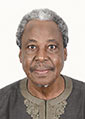Meet Inspiring Speakers and Experts at our 3000+ Global Conference Series LLC LTD Events with over 1000+ Conferences, 1000+ Symposiums
and 1000+ Workshops on Medical, Pharma, Engineering, Science, Technology and Business.

### 4th International Conference on Physics

#### September 17-18, 2018 Berlin, Germany### Biography

Biography: Musa D Abdullahi

### Abstract

This paper assumed that the charge and mass of a particle are independent of its speed relative to an observer. A moving
particle of charge Q and mass m with an electrostatic field Eo at an angle θ to the direction of speed v is considered. The
intrinsic energy of the particle is contained in its electrostatic field. The magnetic field generated takes no energy. It is shown
that, as a result of aberration of electric field Eo, becomes a dynamic electric field Ev displaced by aberration angle α from
the stationary position. Equating the difference between the energy of dynamic field Ev and the energy of electrostatic field
Eo, with the kinetic energy ½ mv2 of the particle, gives a mass-energy equivalence law as E = ½ mc2. It is also shown that a
charged particle moving at time t with acceleration dv/dt produces a reactive electric field Ea = -μoεoφ(dv/dt), where μo is the
permeability and εo the permittivity of space and φ the potential at a point due to the charge. It is proposed that Ea acts on the
same charge Q producing it, to create a reactive force equal and opposite to the accelerating force, so that EaQ = -μoεoφQ(dv/
dt) = -2Eμoεo(dv/dt) = -m(dv/dt), where E = φQ/2 = ½ mc2 is the electrostatic energy and c2 = 1/μoεo, c being the speed of light.
The reactive field Ea explains the cause of inertia of a body as an electrical effect in the body.